# NEET Physics Current Electricity Questions Solved

1.

2.

3.

4.  $\mathrm{Zero}$

Complete Question Bank + Test Series
Complete Question Bank

Difficulty Level:

In the circuit shown in figure find the value of r(internal resistance of the cell) for which power transferred by the cell is maximum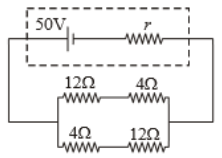1.

2.

3.

4.

Complete Question Bank + Test Series
Complete Question Bank

Difficulty Level:

If the voltage across a bulb decreases by 1%, then the percentage change in its power output is

1.  1%

2.  4%

3.  2%

4.  5%

Complete Question Bank + Test Series
Complete Question Bank

Difficulty Level:

1.

2.

3.  $32\mathrm{\Omega }$

4.

Complete Question Bank + Test Series
Complete Question Bank

Difficulty Level:

1.

2.

3.

4.  $20\mathrm{\Omega }$

Complete Question Bank + Test Series
Complete Question Bank

Difficulty Level:

In the circuit shown in the figure, the current supplied by the battery is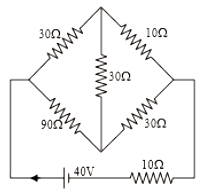1.  2A

2.  1A

3.  0.5A

4.  0.4A

Complete Question Bank + Test Series
Complete Question Bank

Difficulty Level: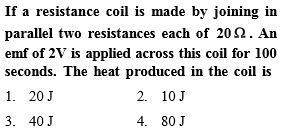Complete Question Bank + Test Series
Complete Question Bank

Difficulty Level:

1.

2.

3.

4.

Complete Question Bank + Test Series
Complete Question Bank

Difficulty Level:

1.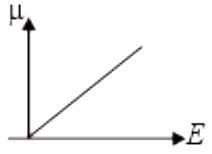2.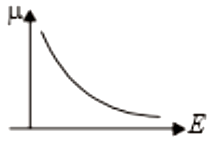3.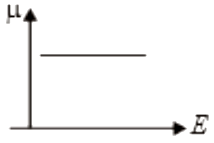4.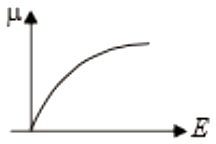Complete Question Bank + Test Series
Complete Question Bank

Difficulty Level:

the equivalent resistance across AB is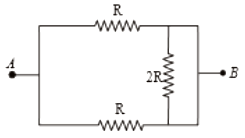1.  2R

2.  R/2

3.  4R/3

4.  3R/2